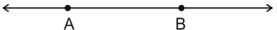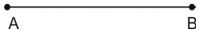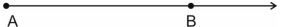### Solutions - Revision Notes

CBSE Class 09 Mathematics

Revision Notes
CHAPTER – 6
LINES AND ANGLES

1. Basic Terms and Definitions
2. Intersecting Lines and Non-intersecting Lines
3. Pairs of Angles
4. Parallel Lines and a Transversal
5. Lines Parallel to the same Line
6. Angle Sum Property of a Triangle

(1) Point - We often represent a point by a fine dot made with a fine sharpened pencil on a piece of paper.

(2) Line - A line is completely known if we are given any two distinct points. Line AB is represented by as  . A line or a straight line extends indefinitely in both the directions.(3) Line segment - A part (or portion) of a line with two end points is called a line segment.(4) Ray - A part of line with one end point is called a ray.It usually denotes the direction of line(5) Collinear points - If three or more points lie on the same line, they are called collinear points, otherwise they are called non-collinear points.

(6) Angle - An angle is the union of two non-collinear rays with a common initial point.

Types of Angles -

(1) Acute angle - An acute angle measure between ${0}^{o}$ and ${90}^{o}$

(2) Right angle - A right angle is exactly equal to ${90}^{o}$

(3) Obtuse angle - An angle greater than ${90}^{o}$ but less than ${180}^{o}$

(4) Straight angle - A straight angle is equal to ${180}^{o}$

(5) Reflex angle - An angle which is greater than ${180}^{o}$ but less than ${360}^{o}$ is called a reflex angle.

(6) Complementary angles - Two angles whose sum is ${90}^{o}$ are called complementary angles. Let one angle be x, then its complementary angle be $\left({90}^{\circ }-x\right).$

(7) Supplementary angle - Two angles whose sum is ${180}^{o}$ are called supplementary angles. Let one angle be x, then its supplementary angle be $\left({180}^{\circ }-x\right).$

(8) Adjacent angles -Two angles are Adjacent when they have a common side and a common vertex (corner point) and don't overlap..

(9) Linear pair - A linear pair of angles is formed when two lines intersect. Two angles are said to be linear if they are adjacent angles formed by two intersecting lines. The measure of a straight angle is 180 degrees, so a linear pair of angles must add up to 180 degrees

(10) Vertically opposite angles - Vertically opposite angles are formed when two lines intersect each other at a point. Vertically opposite angles are always equal.

TRANSVERSAL - A line which intersects two or more given lines at distinct points, is called a transversal of the given line.

(a) Corresponding angles

(b) Alternate interior angles

(c) Alternate exterior angles

(d) Interior angles on the same side of the transversal.

• If a transversal intersects two parallel lines, then

(i) each pair of corresponding angles is equal.

(ii) each pair of alternate interior angles is equal.

(iii) each pair of interior angle on the same side of the transversal is supplementary.

• If a transversal interacts two lines such that, either

(i) any one pair of corresponding angles is equal, or

(ii) any one pair of alternate interior angles is equal or

(iii) any one pair of interior angles on the same side of the transversal is supplementary ,then the lines are parallel.

• Lines which are parallel to a given line are parallel to each other.
• The sum of the three angles of a triangle is $\text{18}{0}^{o}$
• The sum of all angles round a point is equal to ${360}^{\circ }.$
• If a side of a triangle is produced, the exterior angle so formed is equal to the sum of the two interior opposite angles.
• If two parallel lines are intersected by a transversal, the bisectors of any pair of alternate interior angles are parallel and vice-versa.
• If two parallel lines are intersected by a transversal, then bisectors of any two corresponding angles are parallel and vice-versa.
• If a line is perpendicular to one of the given parallel lines, then it is also perpendicular to the other line.